# Working with a two-factor APT model an investor has found two risk factors, X and Y. The investor...

Working with a two-factor APT model an investor has found two risk factors, X and Y. The investor is looking at two stocks, A and B. The expected return on stock A is 12% and that of stock B 10%. The beta (factor sensitivity) of stock A with respect to X is 1 and with respect to Y it is 1/2. The beta of stock B with respect to X is 0 and with respect to Y it is 2. The risk free rate is 4%. What are the risk premia of factors X and Y?

expected return = risk free rate +beta 1*factor 1 + beta 2 *factor 2

for stock A

12=4+1*X+1/2*Y

for stock B

10 = 4+0*X+2*Y

Y = 3

substituting value of Y in stock X equation

12=4+1*X+1/2*3

X = 6.5

##### Add Answer of: Working with a two-factor APT model an investor has found two risk factors, X and Y. The investor...
Similar Homework Help Questions
• ### Consider a three-factor APT model. The factors and associated risk premiums are: Risk Premium (%) Factor Change in gros...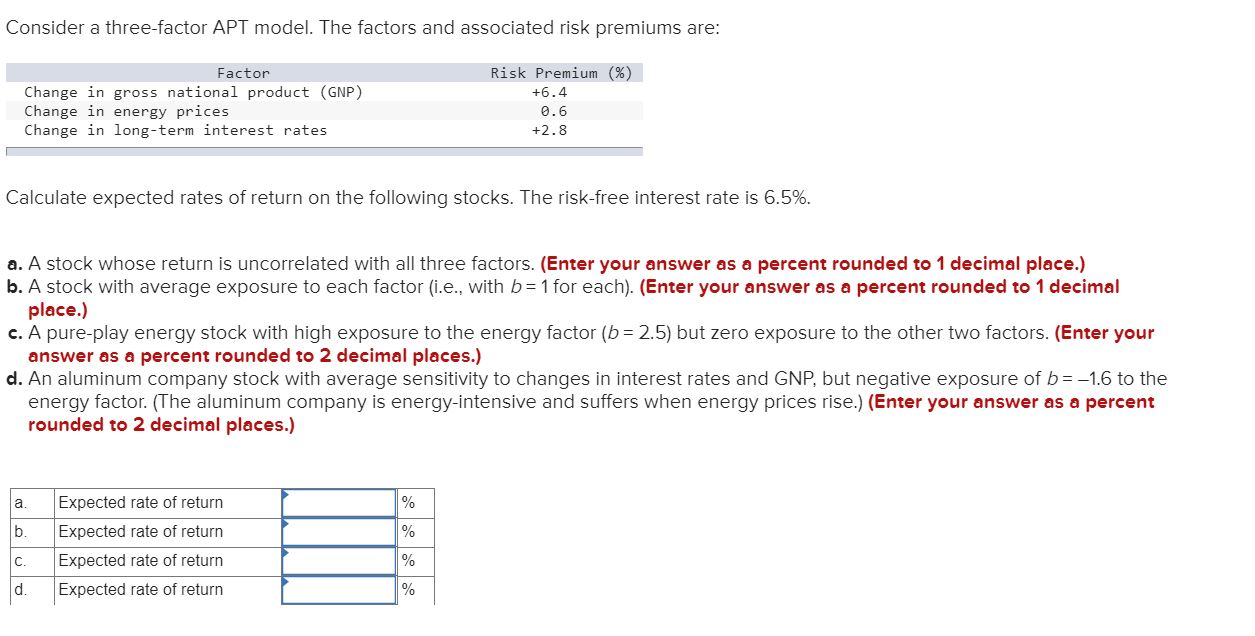Consider a three-factor APT model. The factors and associated risk premiums are: Risk Premium (%) Factor Change in gross national product (GNP) Change in energy prices Change in long-term interest rates +6.4 0.6 +2.8 Calculate expected rates of return on the following stocks. The risk-free interest rate is 6.5% a. A stock whose return is uncorrelated with all three factors. (Enter your answer as a percent rounded to 1 decimal place.) b. A stock with average exposure to each factor...

• ### 2. Suppose there are two independent risk factors governing securities returns according to the two factor...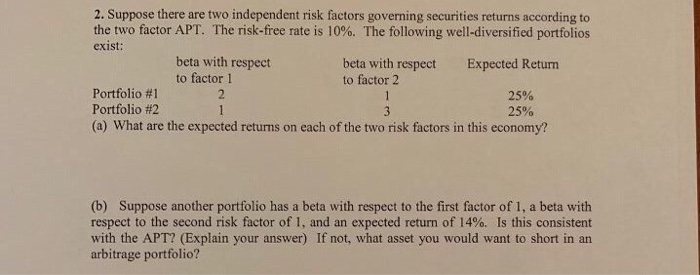2. Suppose there are two independent risk factors governing securities returns according to the two factor APT. The risk-free rate is 10%. The following well-diversified portfolios exist: beta with respect beta with respect Expected Return to factor 1 to factor 2 Portfolio #1 25% Portfolio #2 25% (a) What are the expected returns on each of the two risk factors in this economy? (b) Suppose another portfolio has a beta with respect to the first factor of 1, a beta...

• ### Three-Factor APT Model

I am needing to know how to calculate the following questions in excel.Consider a three-factor APT model. The factors and associated risk premiums are:Factor Risk PremiumChange in GNP 5%Change in energy prices 1Change in long-term interest rates 2Calculate expected rates of return on the following stocks. The risk-free interest rate is 7%.a. A stock whose return is uncorrelated with all three factors.b. A stock with average exposure to each factor (i.e., with b = 1 for each).c. A pure-play energy...

• ### Assume that you are using a two-factor APT model, with factors A and B, to find...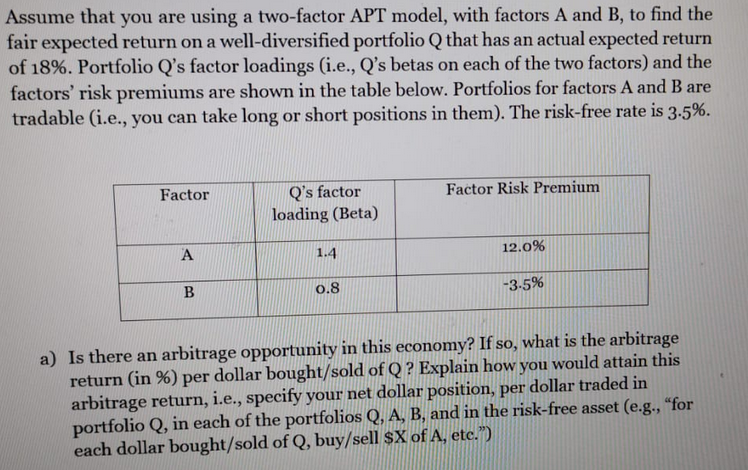Assume that you are using a two-factor APT model, with factors A and B, to find the fair expected return on a well-diversified portfolio Q that has an actual expected return of 18%. Portfolio Q's factor loadings (i.e., Q's betas on each of the two factors) and the factors' risk premiums are shown in the table below. Portfolios for factors A and B are tradable (i.e., you can take long or short positions in them). The risk-free rate is 3.5%....

• ### 9. The Arbitrage Pricing Theory Which of the following statements about the Arbitrage Pricing Theory (APT)...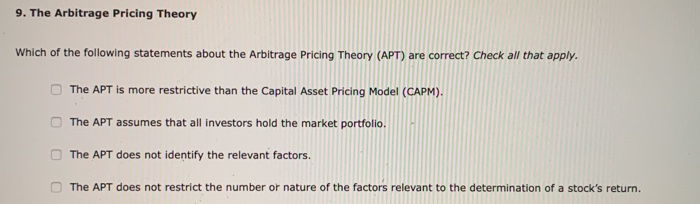9. The Arbitrage Pricing Theory Which of the following statements about the Arbitrage Pricing Theory (APT) are correct? Check all that apply. The APT is more restrictive than the Capital Asset Pricing Model (CAPM). The APT assumes that all investors hold the market portfolio The APT does not identify the relevant factors. The APT does not restrict the number or nature of the factors relevant to the determination of a stock's return. Karine, an analyst at Graffiti Aviation (GA), models...

• ### Please answer Which of the following statements about the Arbitrage Pricing Theory (APT) are correct? Check...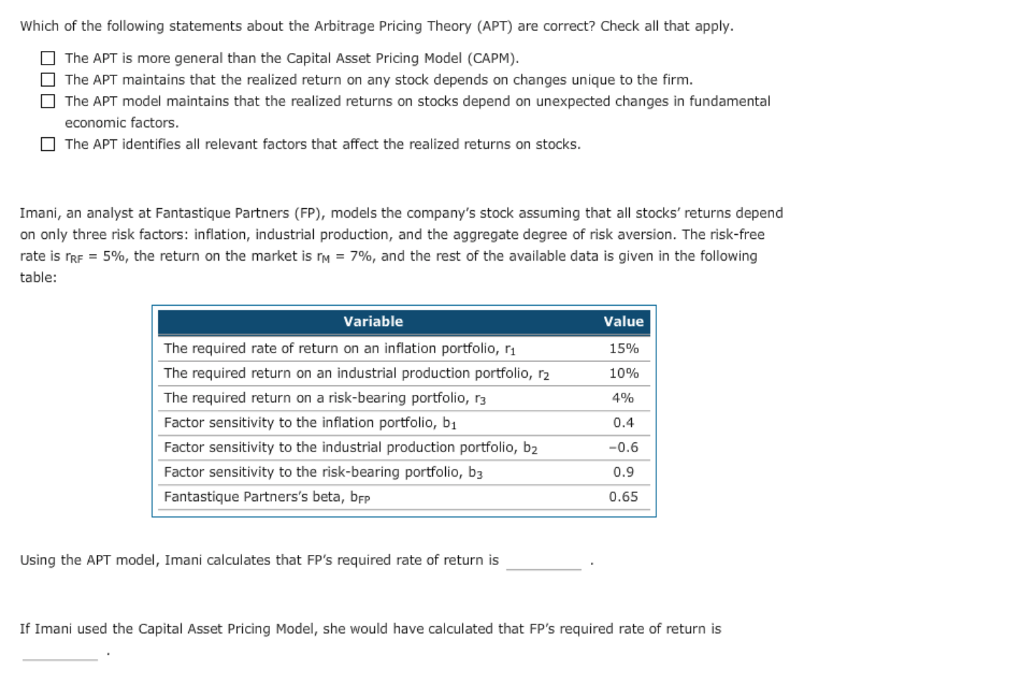Please answer Which of the following statements about the Arbitrage Pricing Theory (APT) are correct? Check all that apply The APT is more general than the Capital Asset Pricing Model (CAPM) The APT maintains that the realized return on any stock depends on changes unique to the firm. The APT model maintains that the realized returns on stocks depend on unexpected changes in fundamental economic factors The APT identifies all relevant factors that affect the realized returns on stocks Imani,...

• ### Consider the following multifactor (APT) model of security returns for a particular stock. Factor Risk Premium...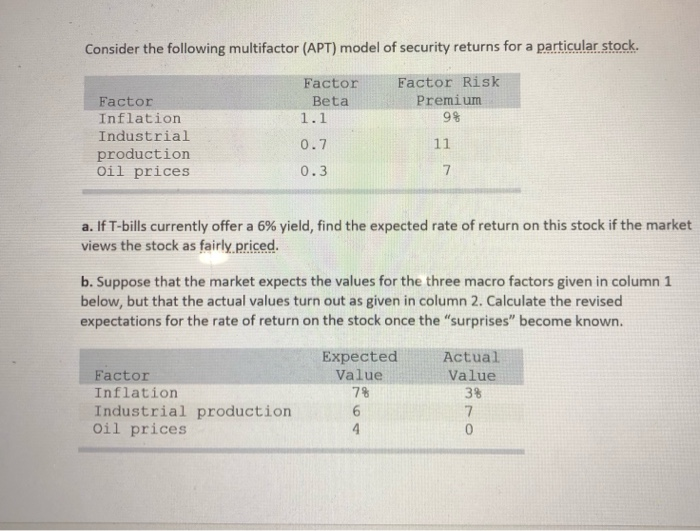Consider the following multifactor (APT) model of security returns for a particular stock. Factor Risk Premium 9% Factor Inflation Industrial production Oil prices Factor Beta 1.1 0.7 0.3 11 a. If T-bills currently offer a 6% yield, find the expected rate of return on this stock if the market views the stock as fairly priced. b. Suppose that the market expects the values for the three macro factors given in column 1 below, but that the actual values turn out...

• ### 9. The Arbitrage Pricing Theory Which of the following statements about the Arbitrage Pricing Theory (APT)...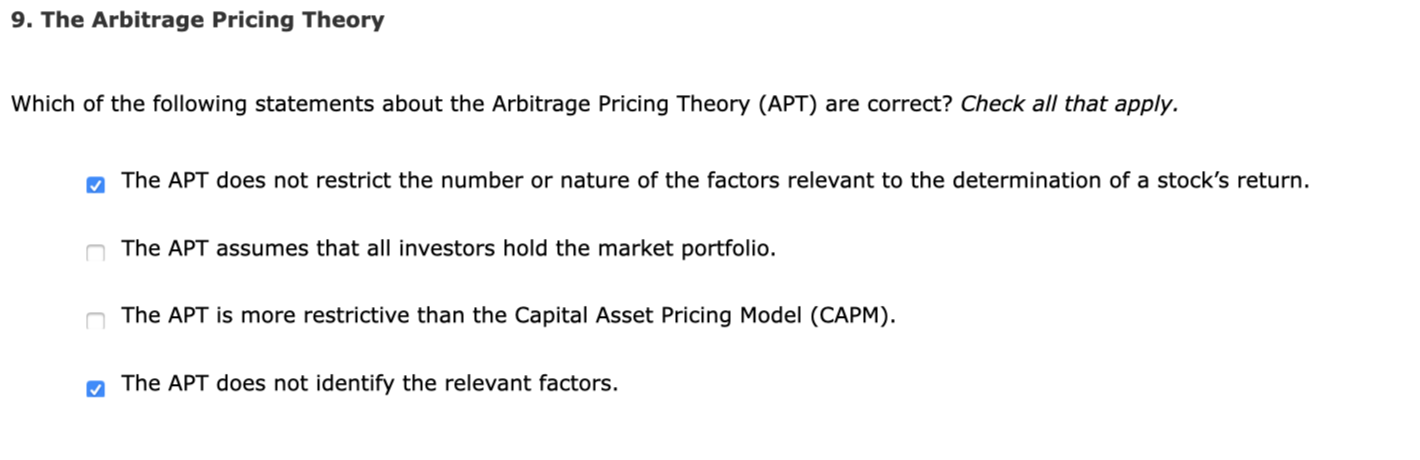9. The Arbitrage Pricing Theory Which of the following statements about the Arbitrage Pricing Theory (APT) are correct? Check all that apply. The APT does not restrict the number or nature of the factors relevant to the determination of a stock's return. The APT assumes that all investors hold the market portfolio. The APT is more restrictive than the Capital Asset Pricing Model (CAPM). The APT does not identify the relevant factors. Emily, an analyst at PietreDure Prestige (PDP), models...

• ### Consider the following simplified APT model: Factor Expected Risk Premium (%) Market 6.8 ...

Consider the following simplified APT model: Factor Expected Risk Premium (%) Market 6.8 Interest rate −.2 Yield spread 4.6 Factor Risk Exposures Market Interest Rate Yield Spread Stock (b1) (b2) (b3) P .8 −1.4 −.2 P2 1.1 0 .4 P3 .3 1.5 1.2 Calculate the expected return for each of the stocks shown in the table above. Assume rf = 4.0%. (Do not round intermediate calculations. Enter your answers as a percent rounded to 2 decimal places.) Expected return P...

• ### Consider the following simplified APT model: Factor Market Interest rate Yield spread Expected Risk Premium (%)...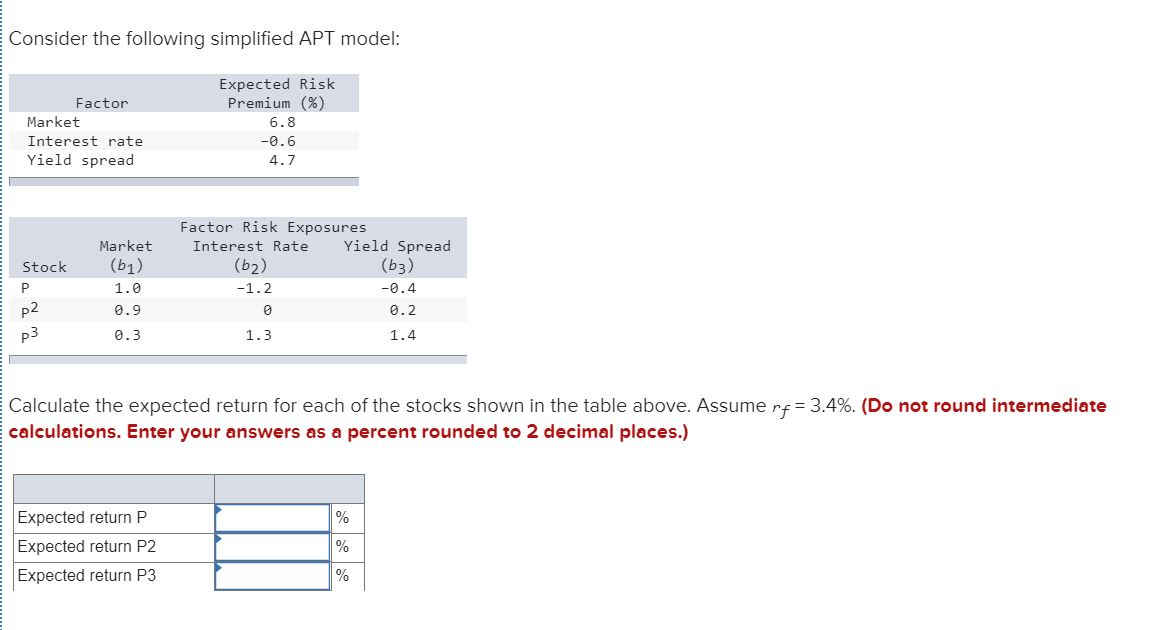Consider the following simplified APT model: Factor Market Interest rate Yield spread Expected Risk Premium (%) 6.8 -0.6 4.7 Stock Market (61) 1.0 0.9 0.3 Factor Risk Exposures Interest Rate Yield Spread (62) (63) -1.2 -0.4 0.2 1.4 1.3 Calculate the expected return for each of the stocks shown in the table above. Assume rf=3.4%. (Do not round intermediate calculations. Enter your answers as a percent rounded to 2 decimal places.) Expected return P Expected return P2 Expected return P3

Need Online Homework Help?